# Nuclear DecayPage 1

#### WATCH ALL SLIDES

Slide 1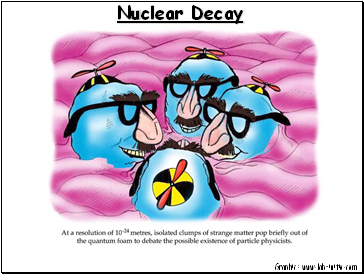## Nuclear Decay

Slide 2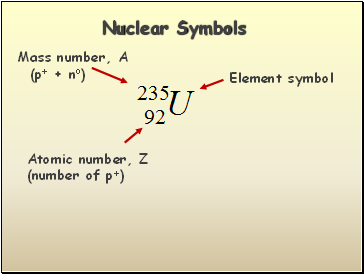## Nuclear Symbols

Element symbol

Mass number, A

(p+ + no)

Atomic number, Z

(number of p+)

Slide 3## Balancing Nuclear Equations

Areactants = Aproducts

Zreactants = Zproducts

235 + 1 = 142 + 91 + 3(1)

92 + 0 = 56 + 36 + 3(0)

Slide 4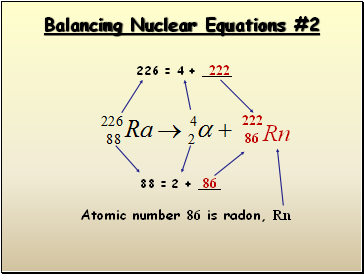Balancing Nuclear Equations #2

226 = 4 +

222

222

88 = 2 + _

86

86

Atomic number 86 is radon, Rn

Rn

Slide 5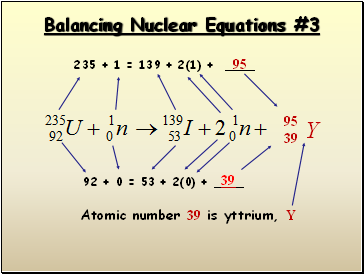Balancing Nuclear Equations #3

235 + 1 = 139 + 2(1) +

95

39

92 + 0 = 53 + 2(0) +

39

95

Atomic number 39 is yttrium, Y

Y

Slide 6## Alpha Decay

Alpha production (a):

an alpha particle is a

helium nucleus

Alpha decay is limited to heavy, radioactive

nuclei

Slide 7Limited to VERY large nucleii.

Slide 8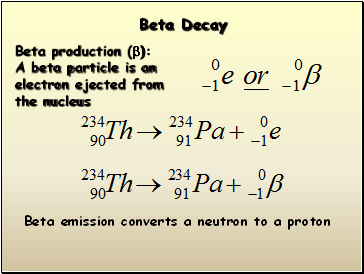## Beta Decay

Beta production (b):

A beta particle is an

electron ejected from

the nucleus

Beta emission converts a neutron to a proton

Slide 9Converts a neutron into a proton.

Slide 10## Gamma Ray Production

Gamma ray production (g):

Gamma rays are high energy photons produced in association with other forms of decay.

Gamma rays are massless and do not, by themselves, change the nucleus

Slide 11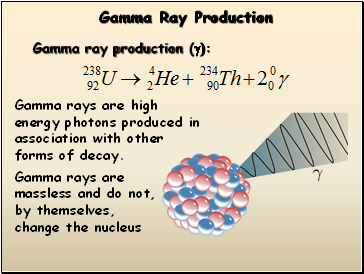Gamma Ray Production

Gamma ray production (g):

Gamma rays are high energy photons produced in association with other forms of decay.

Gamma rays are massless and do not, by themselves, change the nucleus

Slide 12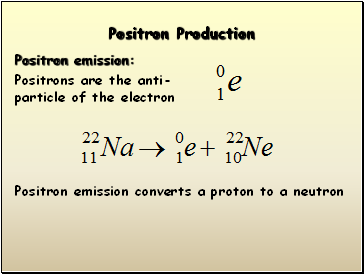## Positron Production

Positron emission:

Positrons are the anti-particle of the electron

Positron emission converts a proton to a neutron

Slide 13## Electron Capture

Electron capture: (inner-orbital electron is captured by the nucleus)

Go to page:
1  2